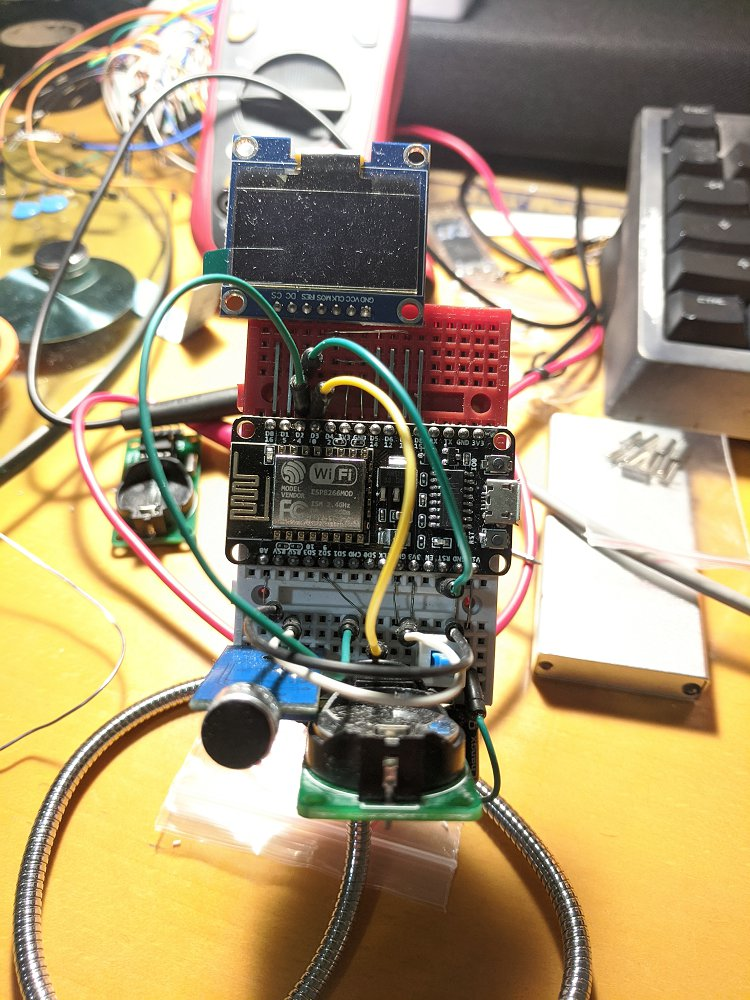# esp8266 wifi小钟# ESP8266 快速改变输出引脚状态

```void ICACHE_RAM_ATTR __digitalWrite(uint8_t pin, uint8_t val) {
if(pin < 16){
if(val) GPOS = (1 << pin);
else GPOC = (1 << pin);
} else if(pin == 16){
if(val) GP16O |= 1;
else GP16O &= ~1;
}
}```

==========更新===========

```//pin最好为常数，以便编译器优化掉判断
#define __digitalWrite(pin,val)\
if(pin < 16){\
if(val) GPOS = (1 << pin);\
else GPOC = (1 << pin);\
} else if(pin == 16){\
if(val) GP16O |= 1;\
else GP16O &= ~1;\
}```